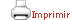Plan docente de la asignatura

 CerrarDatos generales

Nombre de la asignatura: Partículas Elementales

Código de la asignatura: 568428

Coordinación: Joan Soto Riera

Departamento: Departamento de Física Cuántica y Astrofísica

créditos: 6

Programa único: S

 Horas estimadas de dedicación Horas totales 150

 -  Teoría 49.5 -  Teórico-práctica 11
 Aprendizaje autónomo 40

 Recomendaciones

 To have passed an introductory course in particle and nuclear physics, as the one in the physics degree at the UB. To have been exposed to an introductory course of high energy physics and accelerators, as the one in the physics degree of the UB.

 Objetivos de aprendizaje
 Referidos a conocimientos This is an introduction to Modern Elementary Particle Physics.The course is an overview of the field. The course will start with the basic particle physics taxonomy. The role of conservation rulesand symmetries will be discussed. The basics of field theory requiredfor quantification of relativistic processes will be introduced. The three well established  gauge theories: QED, QCD and electroweak interactions will be describedand the basic techniques to evaluate cross sections and decay rates for some processes at first order will be given.

 Bloques temáticos

1. Chapter 1 ``Overview of particle physics’:

*  Elementary particles and interactions; Baryons and mesons;
Weak interactions; More generations.

*  Scalar fields;
Dirac Fermions;
Vectors fields; C, P and T symmetries; Propagators.

3. Chapter 3 "Continuum symmetries in Particle Physics"

*  Symmetry groups and conservation laws; Rotations and angular momentum conservation; Lie groups and Lie algebras; Representations of SU(2) and SU(3).

4. Chapter 4 "The quark model and effective theories of hadrons"

*  Internal symmetries and classification of hadrons; Non-relativistic quark model; The linear sigma model; The non-linear sigma model.

5. Chapter 5 ``QED for leptons"

*  Electromagnetic
interaction as a U(1) (Abelian) gauge theory (QED);
Calculation of scattering amplitudes
and cross sections at tree level for several processes in QED (
e- mu- --> e- mu- , e+e- -->mu+mu- ,e-e- --> e-e-, e- gamma --> e- gamma). Mandelstam variables. Helicity conservation at high energies.

6. Chapter 6 ``QED and the structure of hadrons"

*  Concept of form factors; e-p -->e-p elastic scattering: proton form
factors;  e-p -->e-p elastic inelastic scattering; Bjorken scaling and
quarks; quark distribution functions; the gluons; the QCD Lagrangian.

7. Chapter 6 ``Strong Interactions: Quantum Chromodynamics’

*
Representations of SU(N);
Internal symmetries and classification of hadrons:
SU(2) isospin flavour  and SU(3) flavour.
Evidence of 3 colours: e+e---> hadrons; Lagrangian and Feynman rules for QCD;
q qbar interactions: colour singlet and colour octet configurations;
Asymptotic freedom: perturbative QCD and factorization;
Tests of perturbative QCD: Drell-Yan, e+e--> 2 jets and the spin of
the quark; e+e- --> 3 jets and the spin of the gluon

8. Chapter 7 ``Weak Interactions’

*
Weak decays and parity violation: V-A weak charged currents;
W boson as mediator of weak charged currents; Low energy tests:
muon decay, nuclear beta decay, neutrino decay, neutrino-electron
scattering; fermion mixing matrix; Weak neutral currents: Z0 and the
GIM mechanism; CP violation.

9. Chapter 8 ``Electroweak Unification’

*
Weinberg-Salam Model of
Electroweak Interactions; Spontaneous symmetry breaking: Higgs mechanism;
Masses of the Gauge Bosons and of the Fermions.

10. Chapter 9:``Experimental Techniques in Particle Physics’’

*
Interaction of particles with matter. Types of sub detectors:
calorimeters, tracking and Cherenkov. Accelerators. Measurement of
luminosity. Trigger, event reconstruction and data analysis

11. Chapter 10: "`Example of a HEP experiment: ALEPH’’

*
The Aleph detector. Measurement of the number of light neutrinos. Jets
physics. Search for new physics.

12. Chapter 11 ``Heavy flavour experiments’’

*
The LHCb and BaBar experiments. e+e- vs pp
machines. Flavor tagging. Secondary vertex reconstruction.
Lifetime measurements. Rare decays. CP violation. T violation.

 Theory lectures and resolution of exercises. Exercise sheets to be solved by the students.

 Evaluación acreditativa de los aprendizajes

 The final grade will be based on the homeworks assigned in class and a final work or  exam. The reevaluation will be based on an exam which will take place in June.